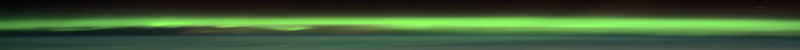The Mission# Instruments

On-board Spin FIT module

The FIT module calculates the E-Field and B-Field vectors by taking 32 points at equal angles and fitting a sine wave least squares fit to the data. The best fit of the data is defined by the formula: A + B*cos() + C*sin(). The module calculates the standard deviation of the fit, and the number of points remaining in the curve. In addition, the FIT module averages the Z-axis data (E and B) and provides in the telemetry.

Data is obtained by the FIT_SAMP routine getting called at one of 32 sectors in the spin. At each sector call, the FIT_SAMP shall call the EFI and FGM modules to obtain samples of E and B, respectively. Since EFI and FGM operate from a variety of time-based sampling frequencies, both sets of electronics have been designed to produce an ever-present 128 Hz signal. EFI and FGM have access to this data and will return the data upon request from FIT.

Given a spin rate of 3 seconds, the use of 128 Hz data for spin fitting puts an apparent phase shift of 360/(3*128) or roughly 0.9 degrees into the results. While this meets the 1.0 degree requirement, the phase shift correction can be determined on the ground using the spin pulse time data relative to the 1Hz tick which is the basis of the 128 Hz data.

Detailed information on the FIT module and spin-fit processing is available in the Flight Software Documents [FIT Module] and [spin-fit processing] .

 Development Institution: Space Science Laboratory (SSL) University of California, Berkeley FSW Lead Peter Harvey (UC Berkeley)